# 第一章 开关理论基础

## 1.2 数制与码制

### 1.2.2 进位计数制的相互转换

• 10进制转其他进制（以10进制转8进制为例）
1. 在整数部分采用除8取余的方式进行，除得的余数是对应8进制数的倒序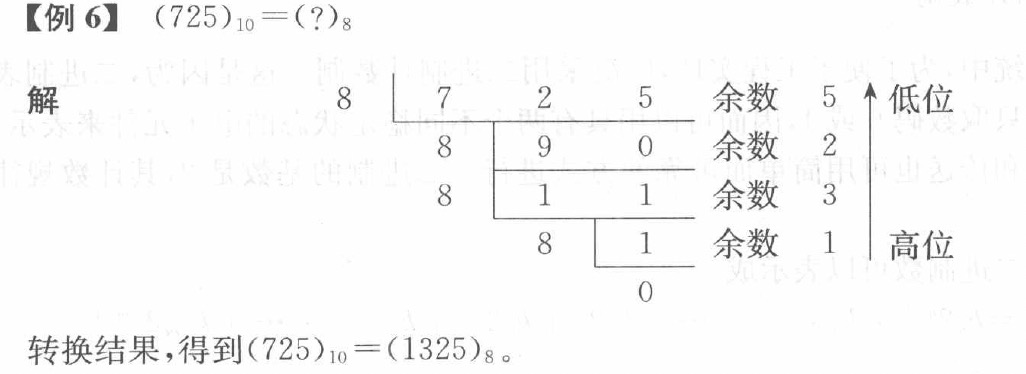2. 在小数部分采用乘8取整的方式进行，乘得的整数部分是对应8进制小数的正序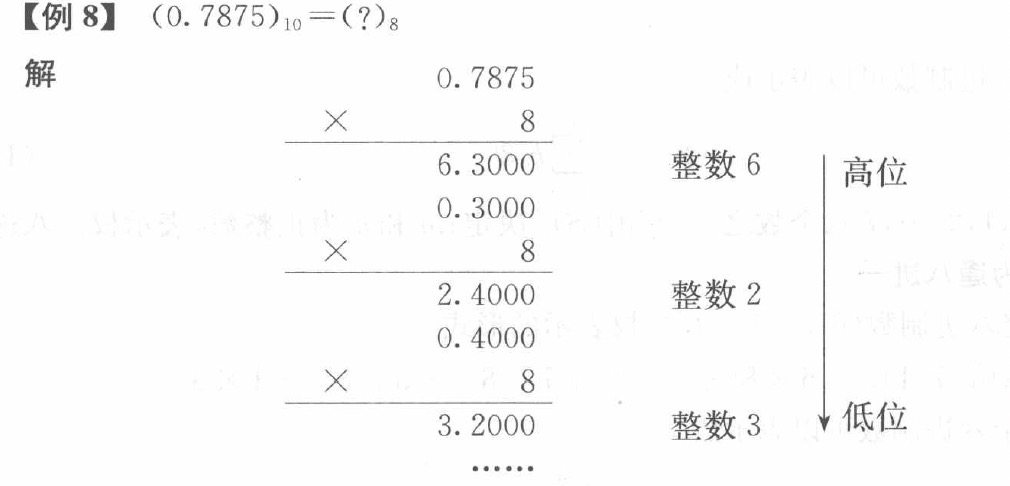• 其他进制转10进制：按照权值相加法即可。
• 八进制，十六进制转二进制：一个八进制数刚好对应3位二进制数，一个十六进制刚好对应4位二进制数。
• 二进制转八进制，十六进制：在整数部分的头部和小数部分的尾部补0，然后按照3个或4个一组转化为相应的八进制或十六进制数。

### 1.2.3 二进制编码

1. 二进制码（值域：0~15）
• 自然二进制码：即最正常的二进制数，按照权值进行计算。
• 循环码：任何相邻的两个码字（如6和7）之间，仅有一位代码不同，其他位代码相同。循环码的编码不是唯一的，4位循环码就有很多种。下图所示为比较基本的一种。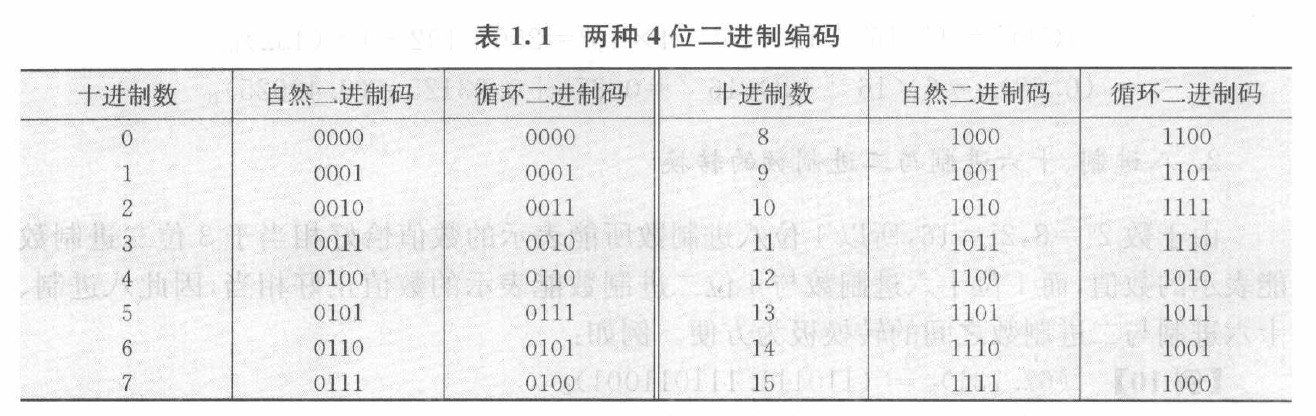2. 二-十进制码（BCD码，值域：0~9）
• 8421码：把0~9用自然二进制码（0000~1001）表示出来，每四位为一个10进制数。
• 5421码：这种编码的显著特点是最高位先连续5个1后连续5个0。
• 2421码：这种编码的0~4与8421码相同，特点是每位取反后刚好是对9的补码。
• 余3码：在8421码的基础上加上0011，特点是执行十进制数相加时能正确的产生进微信号。
• 格雷码：与循环码性质一样，但值域是0~9。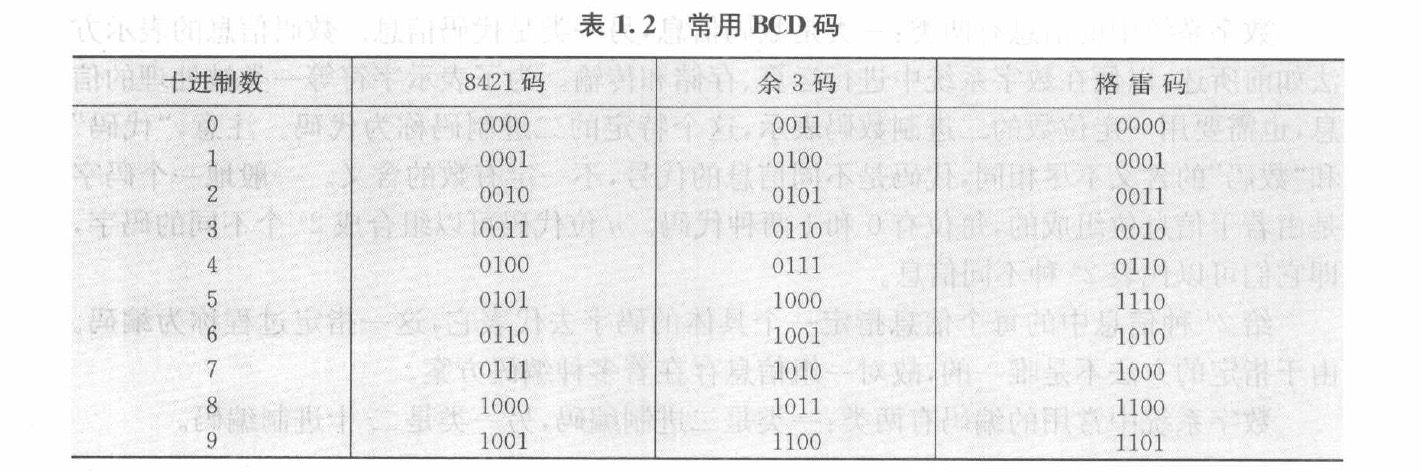## 1.3 逻辑函数及其描述工具

### 1.3.4 正逻辑，负逻辑，三态门

• 如果把门电路的输入，输出电压的高电平赋值为逻辑“1”，低电平赋值为逻辑“0”，这种关系称为正逻辑关系。反之，称为负逻辑
• 三态门的输入有两个：使能端和输入端；输出端有三种情况：逻辑1，逻辑0，高阻抗。当使能端有效（逻辑1）时，输出状态取决于输入端；无效（逻辑0）时，输出端呈现高阻抗状态，意味着输出与后面的电路断开。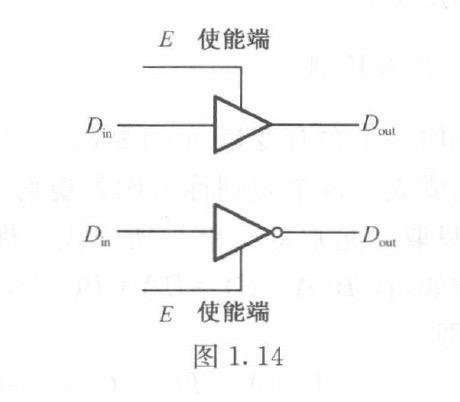## 1.4 布尔代数

### 1.4.1 布尔代数的基本定律

• 分配律
1. $A(B+C)=AB+AC$;
2. $A+BC=(A+B)(A+C)$。
• 吸收率
1. $A+AB=A$;
2. $A(A+B)=A$;
3. $A+\overline{A}B=A+B$
4. $(A+B)(A+C)=A+BC$

## 1.5 卡诺图

### 1.5.1 卡诺图的结构与特点

1. 逻辑函数的最小项表达式
• 一个逻辑函数，如果有$n$个逻辑变量，则有$2^n$个最小项。例如，3个变量的最小项有$\overline{A}\overline{B}\overline{C},\overline{A}\overline{B}C,\cdots,ABC$。可以把他们从0~7编码分别记为$m_0,m_1,\cdots,m_7$。
• 任何一个逻辑函数可以化成一种典型的表达式（相当于离散数学中的主析取范式），这种典型的表达式是一组最小项之和，称为最小项表达式
2. 卡诺图的结构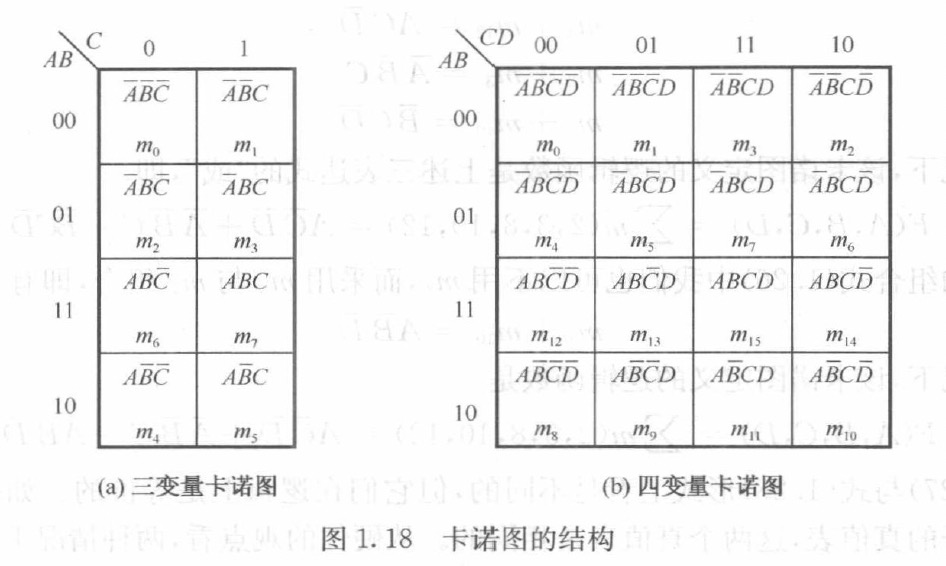注意 卡诺图的横轴和纵轴都采用循环码进行编码，不能随意调换顺序。
3. 卡诺图的有用组合
• 在卡诺图中几何相邻的2个，4个，8个元素，也是逻辑相邻的。此外，卡诺图的第一行和最后一行，第一列和最后一列也是逻辑相邻的。

### 1.5.2 用卡诺图简化逻辑函数

1. 用卡诺图简化逻辑函数的规则和步骤

1. 将逻辑函数表示在卡诺图中
2. 识别围圈8方格的组合，如果不行进行3.。
3. 识别围圈4方格的组合，如果不行进行4.。
4. 识别围圈2方格的组合。
5. 把不能和其他方格组合的一个方格单独围圈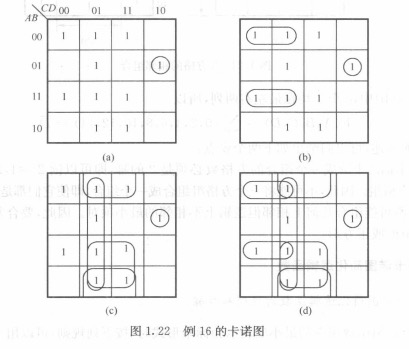2. 具有无关项的化简

• 无关项$\phi$是为有关项服务的，如果一个围圈里围住的全是$\phi$，这个围圈就没有意义。但即使是为了一个有意义的项，也可以为了它围住任意多的$\phi$。
• 在不同的围圈里，相同位置的$\phi$取值可以不同。

# 第二章 组合逻辑

## 2.1 组合逻辑分析

### 2.1.5 组合逻辑中的竞争冒险

• 在组合逻辑中，当逻辑门有两个互补输入信号同时向相反状态变化时，输出端可能会产生过渡干扰脉冲的现象称为竞争冒险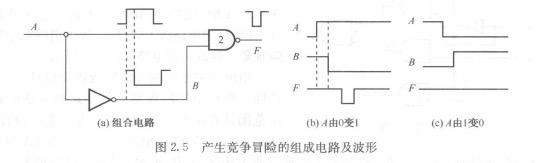• 如图所示，$A$由0变1时，$\overline{A}$会在一个延迟后由1变0。所以存在一个短时间$A$和$\overline{A}$均为1，此时与非门输出0。但是按照逻辑分析，与非门的输出应该恒为1。
• 并不是所有情况下都会出现这种状况，如$A$由1变0时，即使$\overline{A}$的变化有延迟，那也不过是出现了$A$和$\overline{A}$均为0的情况，并不会影响与非门恒为1的输出。
• 解决方案
1. 加选通脉冲：电路的输入发生变化时不立刻输出，在等待一段时间电路稳定后再输出。用选通脉冲$P$实现这一点，平常选通脉冲$P=0$，要输出时$P=1$。
2. 修改逻辑设计：通过构造一些特殊的逻辑，消除竞争冒险（比较费劲，主要是要理清逻辑关系，在稍微复杂一点的电路里面很难看出来）。但其实只要在一开始设计的时候，保证电路中各个门的输入端在信号转换时只有一个变量改变状态就可以了。

## 2.2 组合逻辑设计

• 组合逻辑设计步骤：
1. 根据文字描述的要求列出真值表；
2. 画出卡诺图进行化简；
3. 写出逻辑函数表达式；
4. 根据逻辑函数表达式，搭建组合逻辑电路图。
• 与非门电路：把与或门取两次非。

## 2.4 数据选择器与分配器

### 2.4.1 数据选择器

• 数据选择器是一种多路输入，单路输出的标准化逻辑构件。其输出等于哪一路输入取决于控制信号。如下图，$S_0$和$S_1$的4种真值指派分别对应输出等于$D_0$~$D_3$。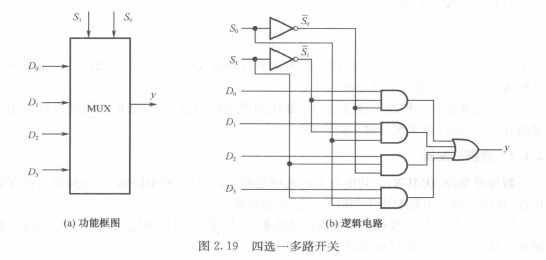### 2.4.2 数据分配器

• 数据分配器的功能与多路选择器相反，它是一种单路输入、多路输出的逻辑构件，从哪一路输出则取决于当时的地址控制端输入。如下图，$S_0$和$S_1$的4种真值指派分别对应输出的线路是$f_0$~$f_3$，不输出的线路为默认值1。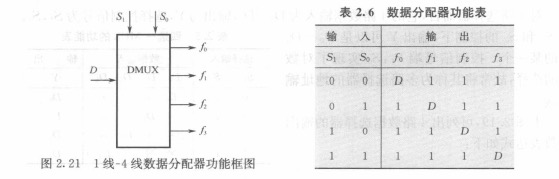## 2.5 编码器和译码器

### 2.5.1 译码器

• 实现译码功能的组合逻辑电路称为译码器，他的输入是一组二进制代码，输出是一组高低电平信号。每输入一组不同的代码，只有一个固定模式的输出组合，这个固定模式的输出组合中只有一个输出呈现有效状态。
1. 3线-8线译码器和2线-4线译码器：
例：输入为101，经过3线-8线译码器译码后输出为11111011。对于101这个输入，输出一定是这样的，而且只有$\overline{Y_5}$这一个输出是有效的。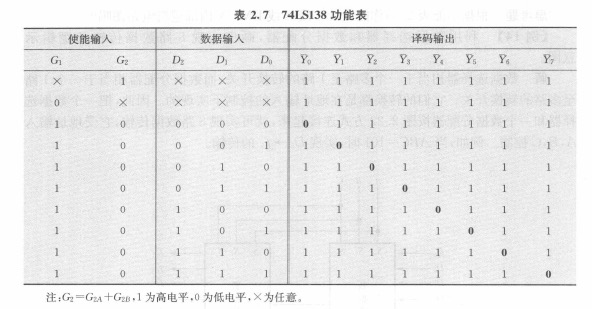2. 七段数字译码显示系统
原理比较简单，如下图所示，但要注意是共阳极还是共阴极。如果是共阴极，则输出为1的二极管亮；如果是共阳极，输出为0的二极管亮。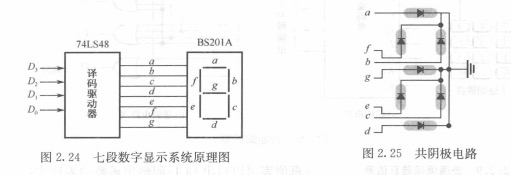### 2.5.2 编码器

1. 普通编码器：
输入是$I_1$~$I_9$中的一个（表示0~9），输出是用$D_0$~$D_3$表示的BCD码。但是如果有两个输入同时有效，输出就会混乱。
2. 优先编码器：
在普通编码器的基础上进行改进，避免输出混乱。比如下图所示的8线-3线优先编码器，当$I_7$有效的时候，不再关心其他的输入，只专注于编码$I_7$。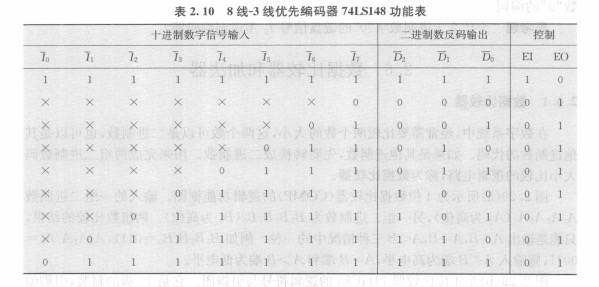## 2.6 数据比较器和加法器

### 2.6.1 数据比较器

• 完成比较两组二进制数码大小比较的逻辑电路，称为数据比较器
以4位比较器74HC85为例，先比较高位，若高位一样，再比较低位，以此类推。原理比较简单，但有趣的是，它们可以通过级联的方式比较更多位数的二进制数，如下图所示：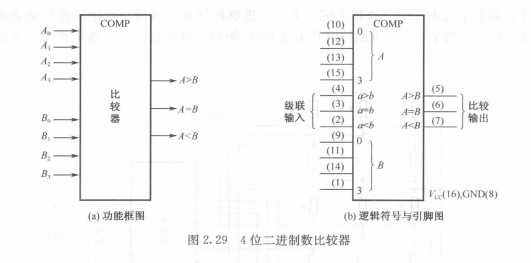先比较高4位，如果高4位不同，则直接输出；若高4位一样，通过级联输入判断低4位。

### 2.6.2 加法器

1. 串行加法器
由多个全加器（FA）串行连接而成。每一个FA有3个输入：加数$A_i$，被加数$B_i$，低位的进位信号$C_{i-1}$；有2个输出：和数$S_i$，向高位的进位信号$C_i$。然后就这样一个一个的把结果加出来。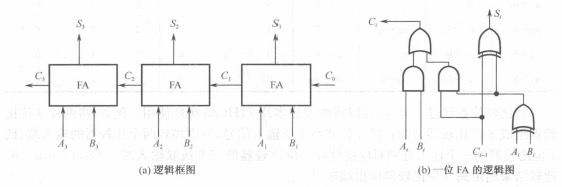2. 并行加法器
为了提高串行加法器的速度，一次性算出来结果。原理是通过归递推公式得到每位结果和输入之间直接的关系，不再依赖上一级的结果。
设$G_i$为进位生成项，$P_i$为进位传递项，即有：
$$G_i=A_iB_i,P_i=A_i\oplus B_i \\ C_i=G_i+P_iC_{i-1}$$
由此递推可得：
$$C_1=G_1+P_1C_0 \\ C_2=G_2+P_2C_1=G_2+P_2G_1+P_2P_1C_0 \\ \vdots \\ C_4=G_4+P_4C_3=\cdots \\$$
各位和的输出为
$$S_i=P_i\oplus C_{i-1}$$
如此一来，便可以不用等待的，一次性的把每一位的结果直接通过输入算出来。

# 第三章 时序逻辑

## 3.1 锁存器

### 3.1.1 锁存器的基本特性

• 锁存器在电路上具有两个稳定的物理状态，所以它们能记忆一位二进制数。它们具有以下特性：

1. 有两个互补的输出端$Q$和$\overline{Q}$；
2. 有两个稳定的状态。通常将$Q=1$和$\overline{Q}=0$称为0状态，$Q=0$和$\overline{Q}$称为1状态；
3. 在输入信号的作用下，锁存器可以从一个状态转移到另一个状态。
• 我们把输入信号发生变化之前的锁存器状态称为现态（PS），用$Q^n$和$\overline{Q^n}$表示；输入信号发生变化之后所进入的状态称为次态（NS），用$Q^{n+1}$和$\overline{Q^{n+1}}$表示。若用$X$来表示输入信号的集合，则有：
$$Q^{n+1}=f(Q^n,X)$$
这个方程被称为锁存器的次态方程，又称现态方程，也称为特征方程

### 3.1.2 基本SR锁存器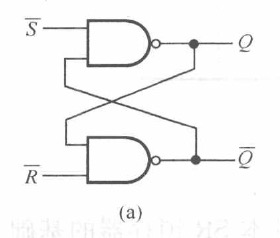1. 若$\overline{S}\overline{R}=00$，两个与非门的输出均为1，也就是说$Q^{n+1}=\overline{Q^{n+1}}=1$，不符合互补输出的条件，此时称锁存器的状态是不稳的。在画卡诺图的时候，这一部分对应的方格就可以用$\phi$表示。
2. 若$\overline{S}\overline{R}=11$，此时锁存器将会延续它的上一个状态。比如上一个状态是1，则$Q^n=1,\overline{Q}=0$。此时若$\overline{S}\overline{R}=11$，可知上面的与非门输出为1，下面的与非门输出为0，刚好与上个状态契合。根据对称性可知，这样的性质对上个状态是0的情况依然适用。
3. 若$\overline{S}\overline{R}=01$，此时先看上面的与非门因为有$\overline{S}=0$的存在所以它一定输出1，然后下面的与非门两个输入都是1，所以输出0。此时锁存器的状态将会无条件变为1。
4. 若$\overline{S}\overline{R}=10$，与上一条同理，锁存器状态变为0。

$$Q^{n+1}=\overline{\overline{S}}+\overline{R}Q^n \\ \overline{S}+\overline{R}=1$$
SR锁存器的状态转移图如下图所示：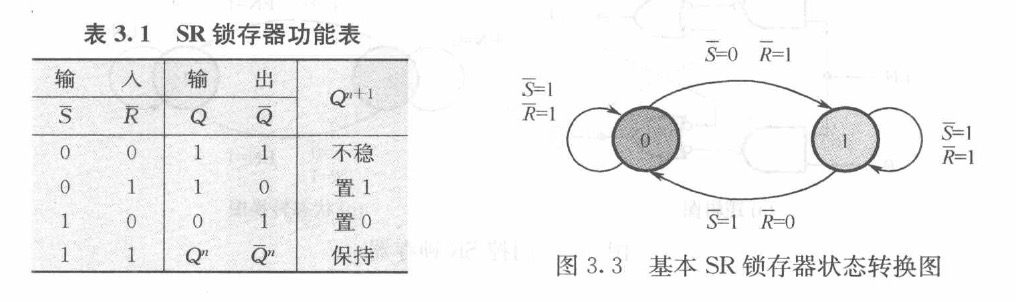### 3.1.3 门控SR锁存器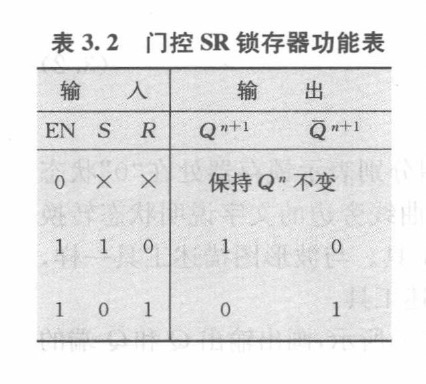• 门控SR锁存器是在普通SR锁存器的基础上改进的产物。当门控信号$EN=1$时，锁存器会发生和普通SR锁存器一样的状态转移；当门控信号$EN=0$时，无论$SR$为什么值，锁存器的状态都不会发生变化。

### 3.1.4 门控D锁存器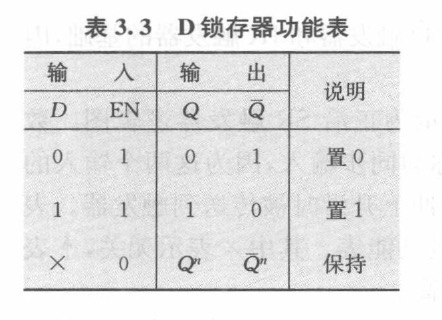• 门控D锁存器进一步改进了门控SR锁存器。通过把$S$和$R$换成$D$和$\overline{D}$，从根本上杜绝了两个输入全为1的情况；同时若将$EN$设置为0，锁存器将依然保留“延续上一状态”的功能。

## 3.2 触发器

• 锁存器虽然可以记忆一位二进制数，但接收的输入数据是在允许使能信号$EN$的控制下进行的。若$EN$保持为1，那么锁存器的状态将会根据输入的变化立刻更改（如1变0或0变1）。但这样就没有节奏的美感，人们为了让锁存器中的状态按照同一节奏，每次在固定的时刻改变状态，推出了边沿方式工作的触发器。
• 触发器与锁存器不同之处在于它把原来的门控信号$EN$替换为了时钟信号$CLK$。每次只会在$CLK$的上升或下降沿的时候改变状态。下图中触发器前有小圆圈的就是在下降沿改变状态，没有小圆圈的就是在上升沿改变状态。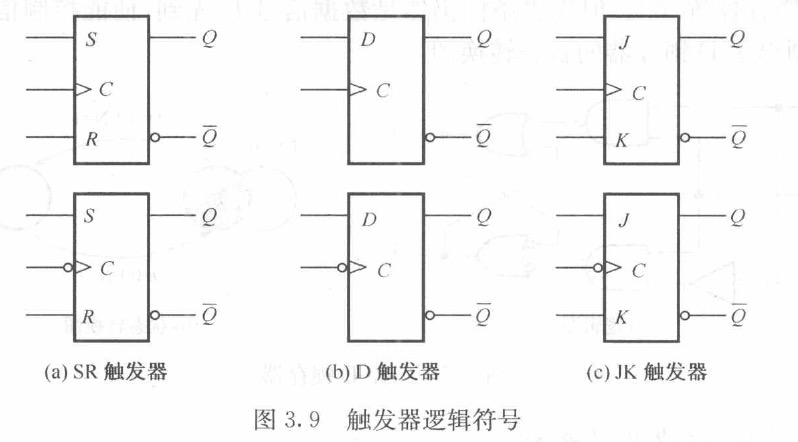### 3.2.1 SR触发器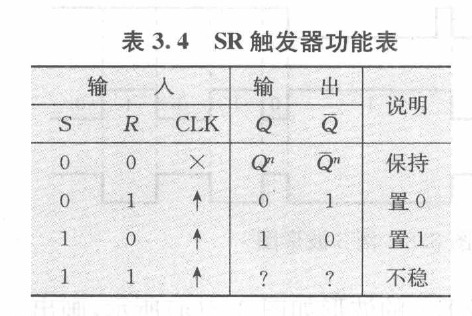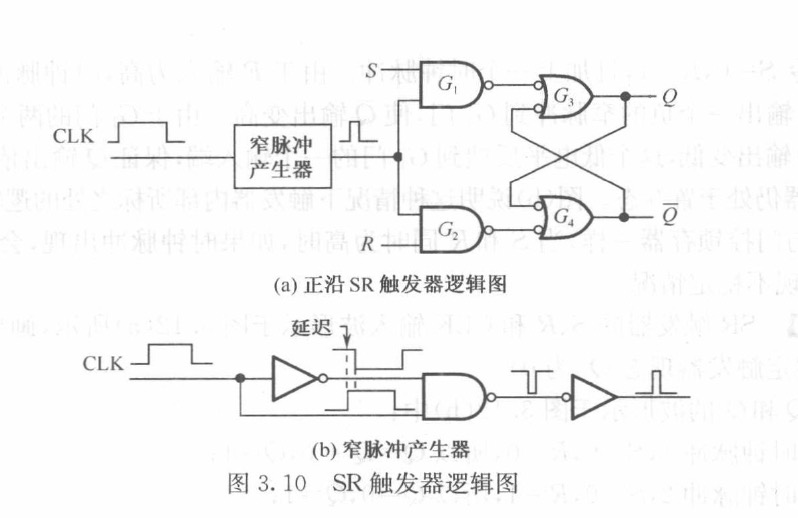• 由上图可知，SR触发器的功能与门控SR锁存器区别不大。但它的电路图比门控SR锁存器有两点改变，这两点改变在从锁存器转化为触发器的工程中几乎是共性的：
1. 门控信号$EN$变为了$CLK$时钟信号；
2. 时钟信号在输入时加了一个窄脉冲产生器。这个器件的作用在于把上升（下降）沿变得更“锋利”，让触发器可以更好的识别上升（下降）沿。

### 3.2.2 D触发器

• D触发器是SR触发器的优化版本，优化原理如同D门控锁存器之于SR门控锁存器。功能比较简单，在此不再赘述。

### 3.2.3 JK触发器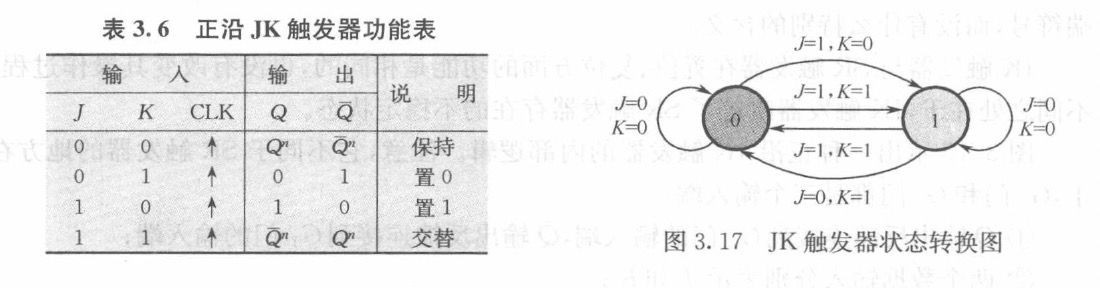• JK触发器和SR触发器在置1，置0和保持这几个方面的功能是完全一样的，但JK触发器优化了SR触发器状态不稳的情况。现在当$JK=11$时，无论触发器的状态目前是什么，都会变成另一个。JK触发器的特征方程非常重要：
$$Q^{n+1}=J\overline{Q^n}+\overline{K}Q^n$$
注意JK触发器的J输入端如果什么都没有接，默认是1；如果输入端接了很多东西，默认是他们取与运算的结果作为输入

## 3.3 寄存器和移位寄存器

### 3.3.1 寄存器

• 课本上介绍了两种寄存器，一种由D锁存器组成，一种由D触发器组成，原理区别不大，这里仅分析D触发器组成的寄存器（74LS374）。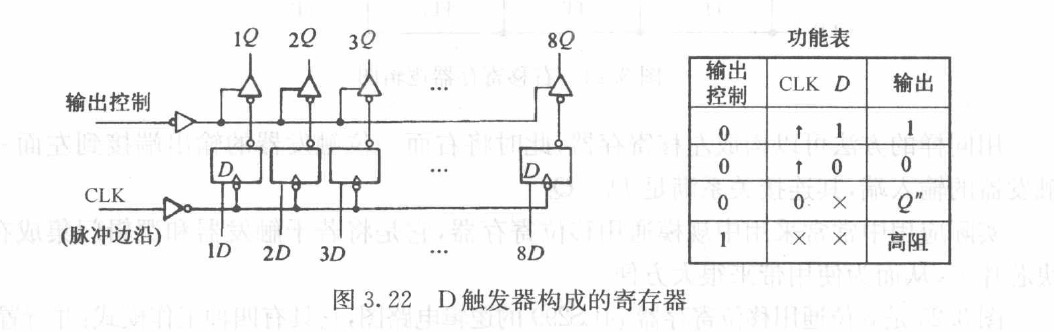当输出控制是0时，寄存器会输出，输出什么取决于当前寄存器自身的状态。如果不在上升沿，则输出上次改变之后完之后的状态；如果在上升沿，那么根据当前的输入D输出改变后的状态。当输出控制是1的时候，寄存器不会输出。在寄存器不输出的时候讨论它的状态变化是没有意义的，因为D触发器的状态只与当前时刻的输入有关，与上一状态无关，如果到了某一个上升沿，输出控制变为0，此时再输出输入的D的值也是来得及的。

### 3.3.2 移位寄存器

• 在时钟信号的控制下，将所寄存的数据向左或向右移位的寄存器称为移位寄存器
• 下图是4位右移寄存器的逻辑图。它的构成很简单，只需把左边一位的输出端接到右边一位的输入端即可，即连接关系满足$D_i=Q_{i-1}$，同时把所有触发器的时钟端连接在一起，用同步脉冲信号控制。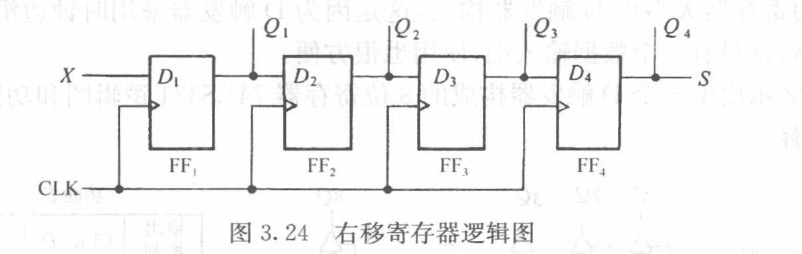• 但实际应用中常常采用中规模通用移位寄存器，如下图所示的74LS299: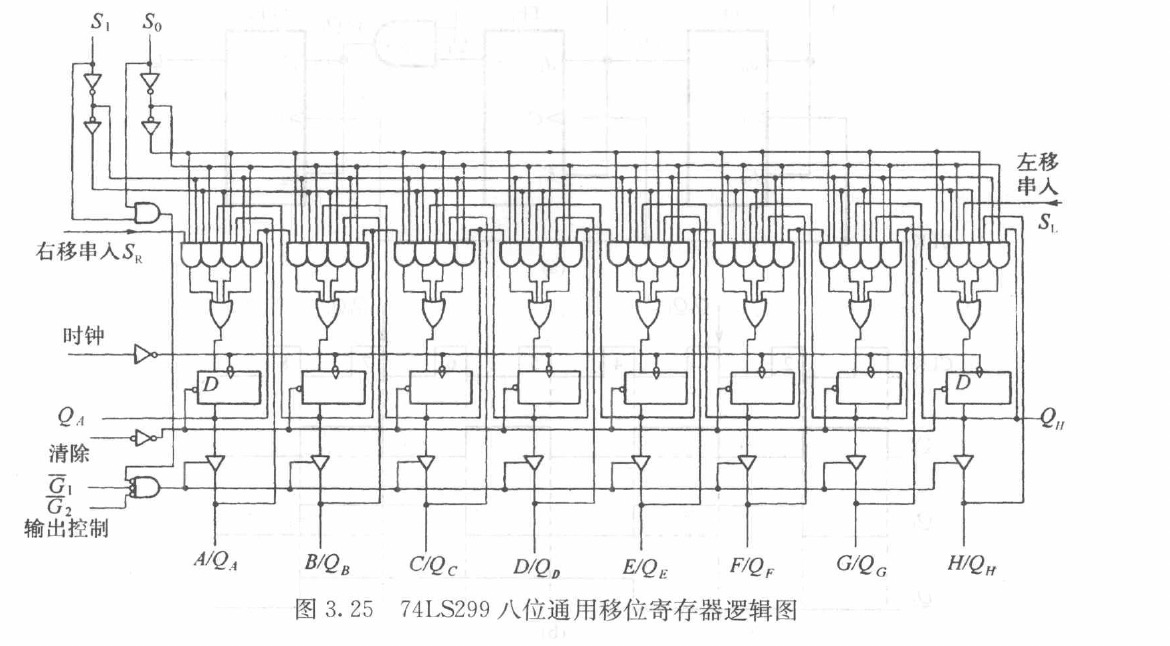这个东西有四种工作模式：并行置数、左移、右移、保持数据，可以实现并入并出，并入串出，串入串出，串入并出操作。

## 3.4 计数器

### 3.4.1 同步计数器

• 同步计数器电路中，所有触发器的时钟都与同一个时钟脉冲源连在一起，每一个触发器的状态变化都与时钟脉冲同步。
1. 用计数方式构成的同步计数器
• 下图所示为3位同步模8计数器逻辑图：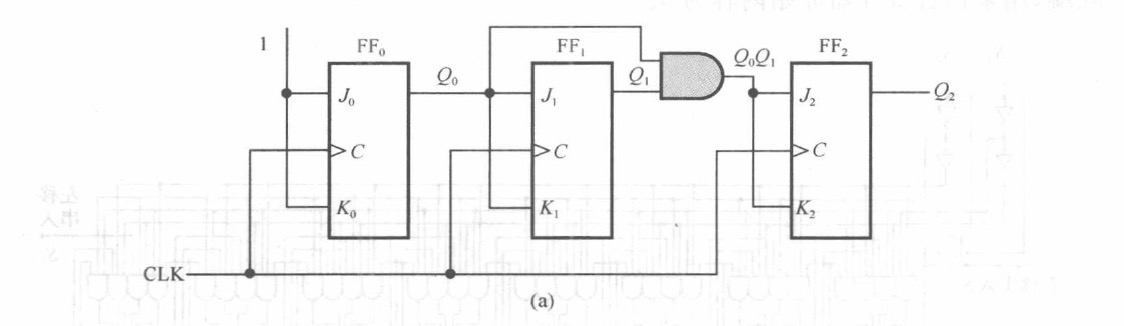由逻辑电路图可知，每次时钟沿到来时$J_0K_0$都为11，也就是说$FF_0$的状态每次都会改变。而$FF_1$只会在每次$Q_0$为1的时候才改变，$FF_2$则只会在$Q_0Q_1=11$时改变。这恰好符合000，001，010…,111，000，…的顺序。
2. 用移位寄存器构成的同步计数器
• 计数器也可以由移位寄存器构成。这时要求移位寄存器有$M$个状态，分别于$M$个计数脉冲相对应，并且不断在这$M$个状态中循环。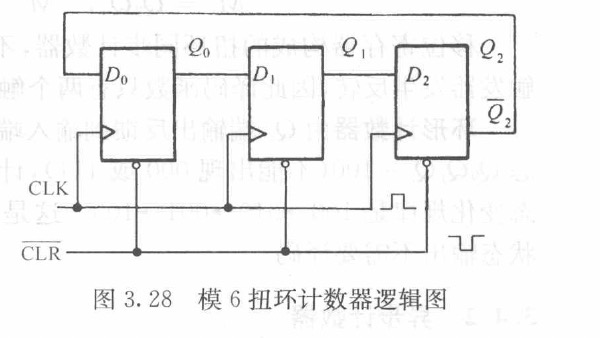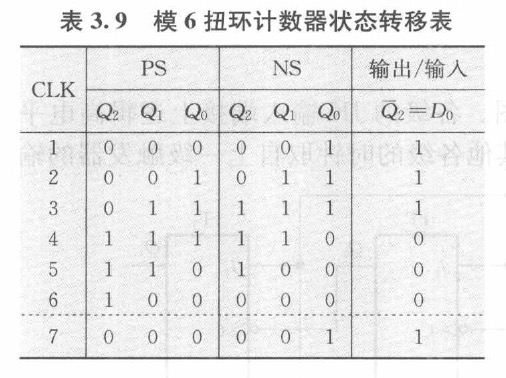• 若最后是$Q_2$反馈，而不是$\overline{Q_2}$反馈，那就需要一个强制端PRE或CLR设置原始状态，因为不能出现000或111。

### 3.4.3 中规模集成计数器

1. 以74LS163为例：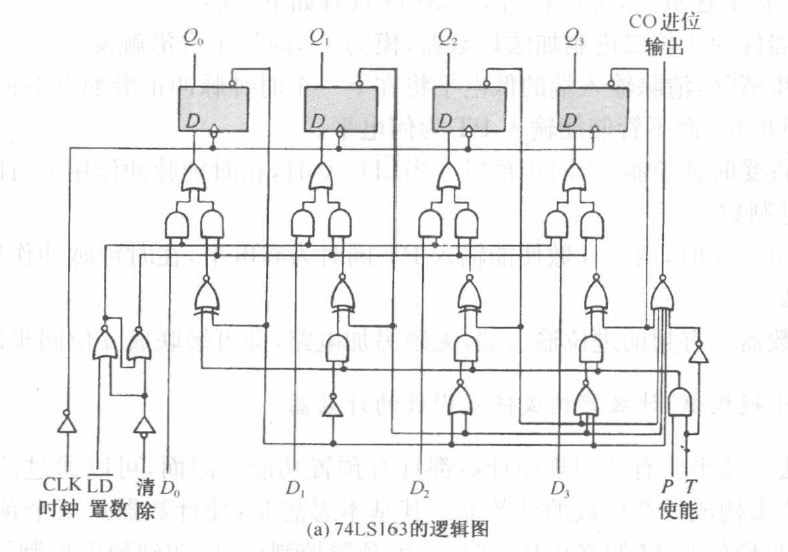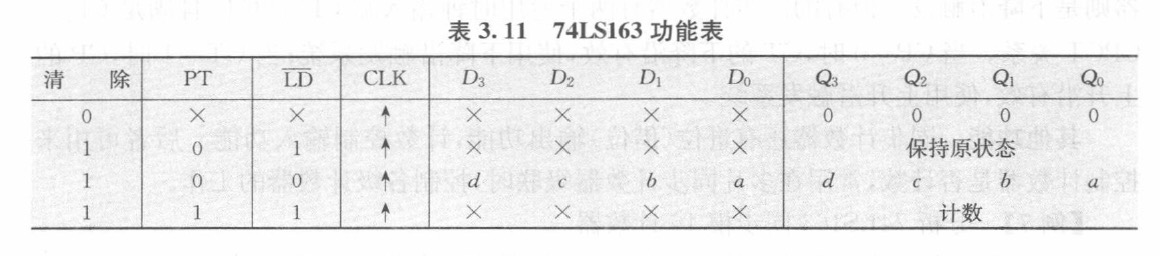在上升沿时，判断计数器执行什么功能：
1. 先看清除端，如果清除为低电平，则无论其他是什么，直接把所有触发器置0；
2. 若清除端为高电平，再看预置端（$\overline{LD}$），若预置端为低电平，则无论其他是什么，都把所有触发器置为预设值dcba。
3. 若清除端为高电平，预置端也为低电平，再看使能端（$PT$）。若使能端为0，则什么都不做，若使能端为1，则计数。
它的逻辑可以用一串if-else的嵌套描述 其他的同步计数器功能类似。
• 值得一提的是，74LS163有一个进位输出，即$D_0$~$D_3$都为1时，再次进行计数，会CO会输出1。这一点可以让很多个74LS163级联成一个可以计数n位的同步计数器。
2. 用中规模IC计数器构成任意模数的计数器
关于下列两个方法，教材上的原话说的非常好，故直接引用。
• 预置法

在实际构成模值为$M$的计数器时，常选用计数达到最大模值的状态为终止状态，因为这样会产生一个进位信号。利用这个进位信号（低电平有效）来作为预置控制信号$\overline{LD}。这时计数器的工作过程为：预置-计数-预置-计数……。$

• 复位法

没有预置功能的中规模计数器都有清零端，因而可通过复位法来构成任意进制计数器。其基本思想是：计数从某个状态开始，到达满足$M$的终止状态时，产生复位信号，使计数器恢复到初始状态，然后重复进行。此时需要外加门逻辑对终止状态进行检测。

3. 用中规模IC计数器级联扩大模数
• 其实只要把上一级的进位信号串入下一级就可以了，可以类比串行加法器。但精彩之处在于可以把IC计数器级联起来之后再用预置法和复位法，真正实现任意模数的计数器。

## 3.6 同步时序逻辑分析

### 3.6.1 同步时序电路的描述工具

• 同步时序逻辑电路按其输入与输出的关系不同，可分为米里型摩尔型两类。当输出是输入变量和状态变量的函数，即输出（$Z$）不仅和该时刻的输入（$X_1,X_2,\cdots,X_n$）有关，而且与电路的现态（Q_1^n,Q_2^n,\cdots,Q_k^n）有关，具有这种特点的同步时序逻辑电路称为米里型时序逻辑电路（判断“101”序列）；而在摩尔型时序逻辑电路中，输出（$Z$）仅仅是状态变量的函数，而和当时的输入无关；或根本就没有$Z$输出，就以电路的状态作为输出（计数器）。

### 3.6.2 同步时序逻辑电路分析的一般方法

• 时序逻辑电路的分析步骤一般概括如下：

1. 根据给定的逻辑图，写出输出函数和激励函数表达式（这就需要对各类触发器的特征方程非常熟悉）；
2. 建立次态表达式及状态转移表；
3. 建立状态表和状态转移图；
4. 分析输出序列与输入序列的关系，说明时序逻辑电路功能。

状态转移表和状态表的区别：
状态表中的状态用$S_0,S_1,\cdots$等标识符表示，表示第几个状态，它具有一定的语义上的含义。
状态转移表中的状态用000,001…表示，其实就是将状态表中的每一个状态都用一个二进制串表示，但此处更强调状态的逻辑含义。

• 下面挑了一道作业题作为例子，分析下图中逻辑电路的功能：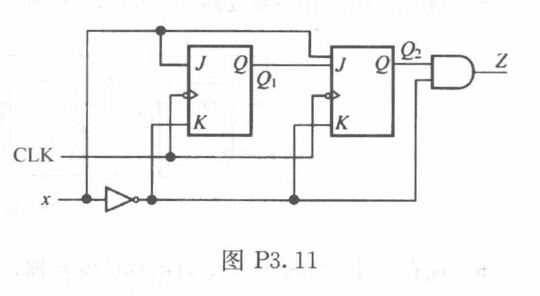• 先画状态转移表，再画状态转移图。中间省略了写表达式和状态表，因为电路比较简单，基本上可以通过状态转移表看出来了。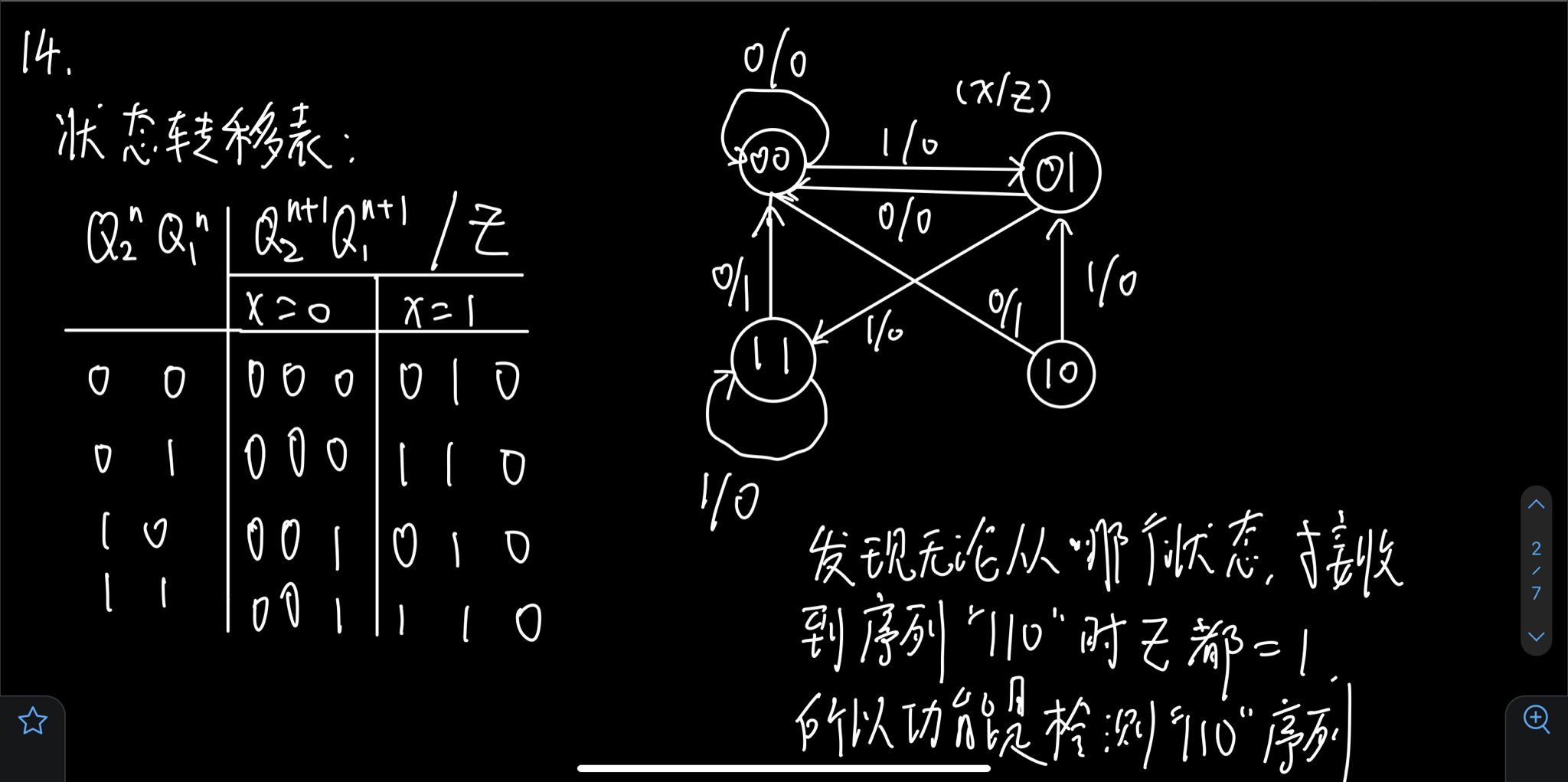### 3.7.1 同步时序逻辑设计方法和步骤

• 同步时序逻辑设计步骤如下（其实就是把分析的步骤反过来）
1. 根据设计要求建立原始状态转移表；
2. 对原始状态表进行简化（如果两个状态在接收相同的输入条件下输出相同，则这两个状态可以归并）；
3. 把每个状态用一个二进制码进行编码；
4. 由状态转移表建立最简激励函数和输出函数表达式（卡诺图），完成组合逻辑设计；
5. 画出逻辑图。

### 3.7.3 状态编码

• 根据对于状态编码（第3步）规则不同，可以将编码方法分为两种（以$S_0$~$S_3$）为例：
1. 一对一法
• 有4个状态，用4个触发器$Q_0$~$Q_3$。
• $S_0$对应$Q_3Q_2Q_1Q_0=0001$;
• $S_1$对应$Q_3Q_2Q_1Q_0=0010$;
• $S_2$对应$Q_3Q_2Q_1Q_0=0100$;
• $S_3$对应$Q_3Q_2Q_1Q_0=1000$;
• 触发器多，逻辑简单
2. 计数器法
• 有4个状态，用2个触发器$Q_2Q_1$
• $S_0$对应$Q_2Q_1=00$;
• $S_1$对应$Q_2Q_1=01$;
• $S_2$对应$Q_2Q_1=10$;
• $S_3$对应$Q_2Q_1=11$;
• 触发器少，逻辑复杂
• 一道作业题，设计与下图对应的电路：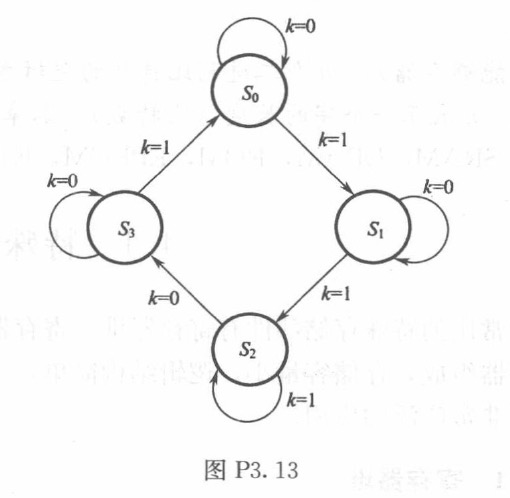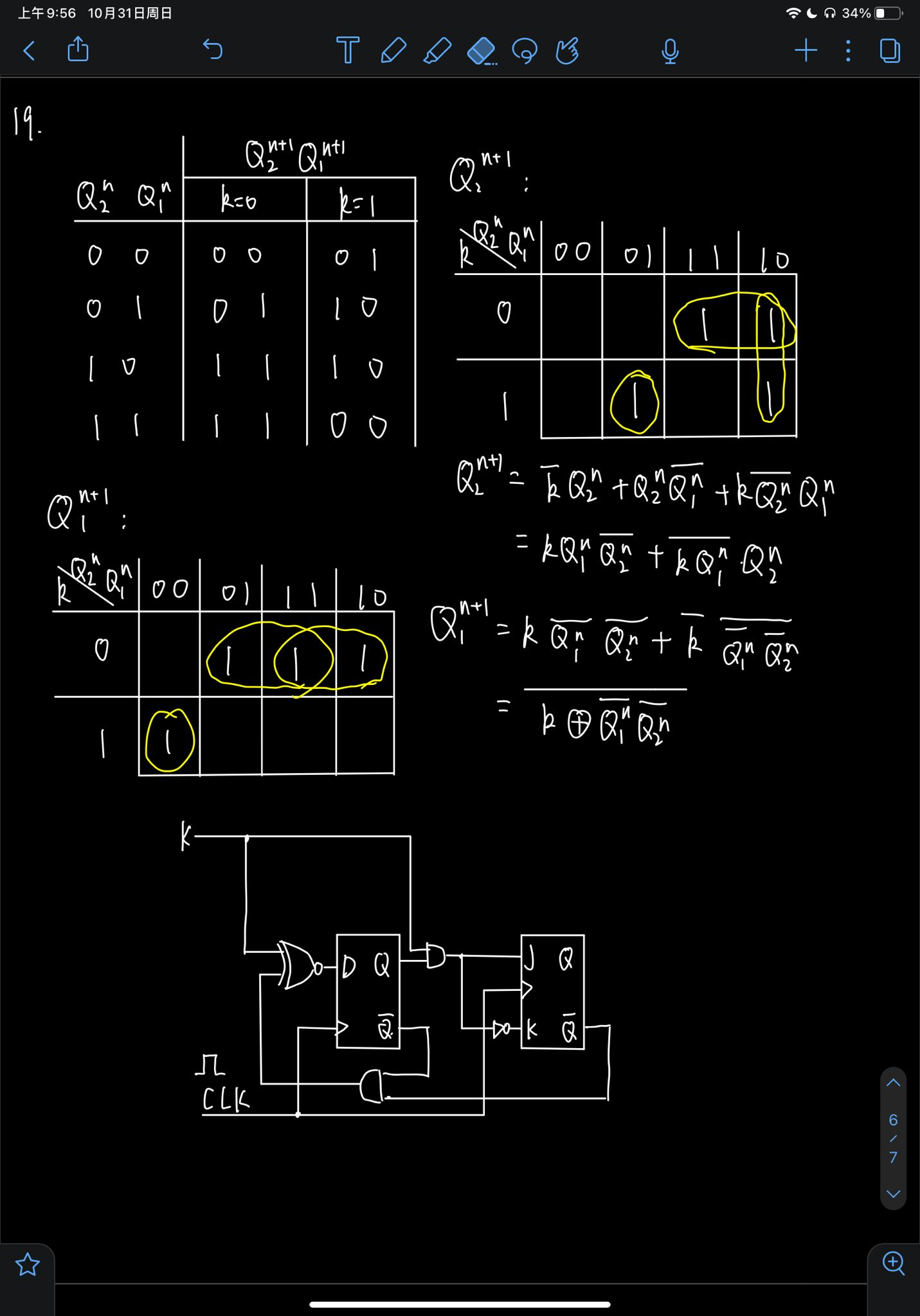https://heyewuyue1.github.io/2021/10/28/digitalLogicSystemMidTerm/

2021年10月28日